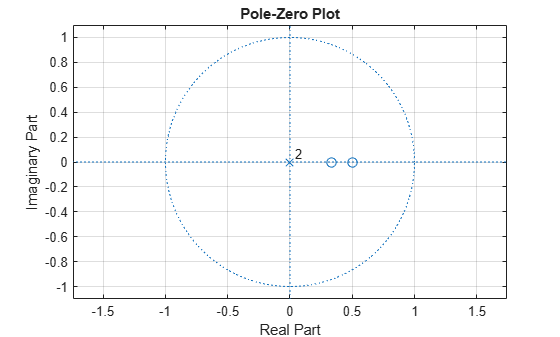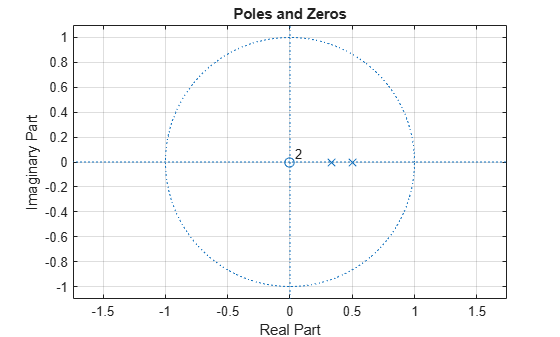# Prediction Polynomial

This example shows how to obtain the prediction polynomial from an autocorrelation sequence. The example also shows that the resulting prediction polynomial has an inverse that produces a stable all-pole filter. You can use the all-pole filter to filter a wide-sense stationary white noise sequence to produce a wide-sense stationary autoregressive process.

Create an autocorrelation sequence defined by

`$r\left(k\right)=\frac{24}{5}\phantom{\rule{0.16666666666666666em}{0ex}}{2}^{-|k|}-\frac{27}{10}\phantom{\rule{0.16666666666666666em}{0ex}}{3}^{-|k|},\phantom{\rule{1em}{0ex}}k=0,1,2.$`

```k = 0:2; rk = (24/5)*2.^(-k)-(27/10)*3.^(-k);```

Use `ac2poly` to obtain the prediction polynomial of order 2, which is

`$A\left(z\right)=1-\frac{5}{6}{z}^{-1}+\frac{1}{6}{z}^{-2}.$`

`A = ac2poly(rk);`

Examine the pole-zero plot of the FIR filter to see that the zeros are inside the unit circle.

```zplane(A,1) grid```The inverse all-pole filter is stable with poles inside the unit circle.

```zplane(1,A) grid title('Poles and Zeros')```Use the all-pole filter to produce a realization of a wide-sense stationary AR(2) process from a white-noise sequence. Set the random number generator to the default settings for reproducible results.

```rng default x = randn(1000,1); y = filter(1,A,x);```

Compute the sample autocorrelation of the AR(2) realization and show that the sample autocorrelation is close to the true autocorrelation.

```[xc,lags] = xcorr(y,2,'biased'); [xc(3:end) rk']```
```ans = 3×2 2.2401 2.1000 1.6419 1.5000 0.9980 0.9000 ```